Don't trust, verify!

## What is Hash Rate Proof?

Hash Rate Proof is a method developed by Slush pool with the aim to prove that the total hashrate of the pool declared by the pool operator is accurate.

## Why is such Proof useful?

Until recently, independent third party verification of hash rate which the pool has been publishing was not possible. Bitcoin miners have had to trust the pool operator to be honest. But we have decided to be even more transparent and start to publish more comprehensive data. Such transparency and more comprehensive data-set will allow everyone to answer the following questions and do some interesting data investigations as well.

• Have you ever wondered if the hash rate of the pool is accurate and that the pool does not cheat the miners on payouts?

• Did you experienced "Bad Luck" period with the pool and questioned the pool operator's honesty?

• Would you like to check the pool "luck" on different difficulty levels?

• Are you interested in quite exact distribution of BIP voting and more to come?

• ## Basic explanation

As mentioned above, we have started to collect more accurate data with certain unique characteristics from our miners. Such data can not be counterfeited because certain amount of work has to be done in order they are generated. You can imagine them as a data (hashes) which did not meet the criteria to be validated as a regular block but were really close with their characteristics to it.

If you analyze such data you can independently verify the total hash rate of the pool. If you are interested in a more detailed explanation, please read the following sections in our manual where you can find a simple and commented python script for reading and validating our published data which will do the math for you. It's main output is an average hash rate of the pool based on our published data. Since the script is an open source, everyone can run it on his/her own computer. Moreover it can be easily analyzed if it does what it claims.

### Data-set publication process and schedule

Firstly, we'll define and publish a number, difficulty limit (currently 10⁶).

When we receive a result of mining work, we'll validate it as usual and if its difficulty is at least the difficulty limit mentioned above, we'll collect it for publication.

Every hour, we'll publish a compressed file with all the collected block candidates from all our servers together, pre-processed for user convenience.

Because we will publish all shares with certain difficulty and above, it can be calculated how much hash rate we really have and is spent on productive mining! It is the same calculation the pool does for estimating each miner's hash rate - only extended to the pool scale.

### Dataset - what kind of data you find there

We'll provide complete block header + merkle branch + coinbase transaction for each collected submission in JSON format. So it can be fully validated by anyone interested. E.g. it can be checked that the difficulty is met, the work was spent on correct prevhashes, ntimes are correct, submissions are unique, etc., all the technical details. The important thing is that it can be seen that the work was done for our pool - by finding "/slush/" marker in coinbase and by coinbase transaction output.

Note: We have defined the difficulty limit in a way which allows us to publish enough data showing quite precisely the total pool's hash rate is and on the other hand not too much data to allow anybody to download it regularly.

## Technical explanation

In order to understand the technical explanation it is useful to understand how the Bitcoin hashing function works, which data the pool collects from its miners, and how the pool is estimating the hash rate of its miners.

Hash Rate Proof of the Pool is analogous to a proof of each miner's hash rate being submitted to the pool. The only difference is who is proving to whom. In the former case, the data are sent from the miners to the pool, while in the latter case the pool operator publishes the data received by miners. The principles of the calculation stay the same.

### Hashing function

Hashing function is in Bitcoin context the double-SHA256 function which takes a block of data and returns (outputs) a number of fixed length with a value from the range 0 to 2²⁵⁶. Even a small change in the block of data (i.e. one bit being changed) will result in a completely different hash output. Every hash value in the output range is generated with the same probability, which is important property of this function.

Each output range value has probability as follows: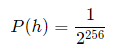### How does the mining and hash rate calculation work?

1. Pool is sending work to miners in the form of almost complete 80B block header templates. The header is missing a small part that needs to be filled in by the "correct" random data. Basically, mining is the process of hashing the block header repeatedly, changing that random data in the block header, until the resulting hash matches a specific target. Such target is given by the difficulty of the network and it is adjusted regularly to keep the block generation time at 10 minutes.

2. If the miner finds the hash lower than 2²⁵⁶2³² (which is once in 2³² tries) it sends 80B header block back to the pool as a result of its work. Such a proof of work is called Share. Based on this information the pool is able to verify that the miner is working and even compute the hashing power of such miner. Lets suppose that the miner sent 100 of such 80B candidates for the block in one minute.

Pool can easily compute that the miner had to try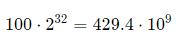hashes on average. Therefore it means that the hash rate of the miner is 7.19 Gh/s.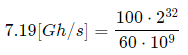The value of 2³² was chosen arbitrarily in the past, but is stable and makes one share well defined. In reality, proof of work value may vary from miner to miner, but it is just an implementation detail. The important thing is that with regular submits and the known limit to the submitted work the pool can compute the number of attempts which the miner had to do in the given time.

3. Once the miner submits the share with a hash value so low that it also meets the stricter limit for the block, the pool publishes the data into the Bitcoin network. A new Bitcoin block is created. Limit for the hashing function result satisfying the block condition is lower by multiple orders. It means that finding such result is much more work intensive. The pool requests data more often in order to make hash rate calculation more precise.

### Dice rolling analogy

Let's imagine a game where players roll a dice repeatedly, trying to roll a valid value on the dice. It is known in advance how many sides N the dice has and values which are counted as valid L (1 ≤ L ≤ N). Moreover it is known how many marks representing thrown valid values are on the paper C. What is NOT known is count of dice throws H, which were necessary to generate marks on the paper. In other words it is NOT known how much work was needed to do the job. However the count of throws can be easily estimated as follows: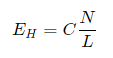Ratio NL can be considered as an average count of throws per one mark. In reality, when C and L are big enough, the EH is not different from real H (their limits are equal). Therefore we can substitute EH with H  in our calculations. In simple terms, if the player rolls long enough and the number of marks is huge, it is easy to determine the number of required tries with arbitrarily small deviation.

And now what matters the most. Mining and Hash Rate calculation for a particular miner is equivalent to the dice rolling analogy with following substitutions:

• Dice = Hashing function

• Dice values1 − N = Hashing function values in the range 0 − 2²⁵⁶⁻¹

• One dice roll = One hashing computation over block candidate header (80B)

• Limit L on the rolled value, when we mark down the value = Number from range 2²⁵⁶2³² determining the probability of work submission

• Mark on the paper = Unique block header with a hash value lower than the limit L

• Number of marksC = Number of 80B block candidates submitted by miner

• Dice rollsH = Count of unique block candidates tries, thus real work

• Guessed number of dice rolls EH = Pool's guess regarding the work done by miner

• The last item in the list above is probably the most important one. Proof of miner's hash rate is observed indirectly by measuring the work that the miner has submitted. The pool can compute the EH easily and therefore knows what is the likely hash rate of the miner. It is crucial for the pool operator that the miner can not cheat its submitted data in any way. The reason is simple, each submitted block represents a unique piece of data. Discovering it requires a certain number of attempts (NL). It can not be altered in any way because of hashing function properties described above. As long as the miner is sending enough results, the pool operator can compute the hash rate of the miner with arbitrary precision.

## The Math Behind the Scenes

Let's define L2²⁵⁶2³²⁺¹⁰ for the entire pool. Each block candidate (80B) received by the pool whose hash value is less than or equal L will be published. (Therefore the pool will publish each 2¹⁰ share on average which means each 2⁴² hash tried). The data is going to be published every hour and the number of published hashes is Q.

The expected hash rate of the pool EHr can be computed easily by using following formula: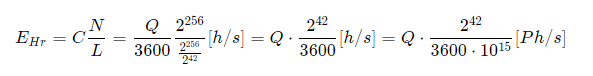where C = Q / 3600 and defines an average number of block candidates per one second and L is an arbitrary set value.

It can be concluded that hash rate is linearly dependent on the number of published block candidates. Which is exactly what might be expected.